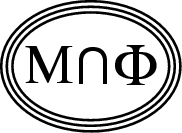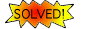Back to
OP page

Combined
List

List by
Contributors

# Why is the Hall Conductance Quantized?

### Abstract   Formulate the theory of the Integer Quantum Hall effect, which explains the quantization of the Hall conductance, so that it applies also for interacting electrons in the thermodynamic limit.Solved by M.B. Hastings and S. Michalakis.
The problem's analysis was aided by advances which were made in the joint works by A. Giuliani, V. Mastropietro and M. Porta, and by S. Bachmann, W. de Roeck and M. Fraas. See J. Avron's summary for a more complete account.

The question as it was originally posed:

The Integer Quantum Hall effect, first observed by K. von Klitzing, is used to determine the fine structure constant with precision that is comparable to the precision one gets from atomic physics. It is also used as a practical and fundamental way to define the Ohm.

It is instructive to look at the experimental data. The graph that looks like a straicase function has remarkably flat plateaus. The ordinates of the plateaus correspond to integer integer multiple of the quantum unit of conductance, and can be measured very precisely.

An intriguing aspect of this phenomenon is that a precision measurement of fundamental constants is carried on a system that is only poorly characterized: Little is actually known about the microscopic details of the system, which is artificially fabricated, and whose precise composition and shape are not known with a precision that is anywhere comparable with the precision that comes out of the experiment.

There are two related but somewhat distinct theoretical frameworks that attempt to answer this question. The problem we pose has to do with their mutual relation, and the extent to which they give a satisfactory answer.

One framework identifies the Hall conductance with a topological invariant: The first Chern number of a certain bundle associated with the ground state of the quantum Hamiltonian. This framework applies to a rather general class of quantum Schrodinger Hamiltonians, including multiparticle ones. It has two principal drawbacks. The first is that it requires an interesting topological structure: It applies in cases where there is a Brillouin zone, and  in  cases where configuration space is multiply connected. The multiple connectivity can be motivated, to some extent, by the experimental setup if one includes the leads that connect to the two dimensional electron gas in the system. This makes the Hall conductance a property of the system and not just of the two dimensional electron gas. The second drawback is that the Chern number is identified with a certain average of the Hall conductance. In some cases this average comes for free, but in general it does not.

A second theoretical framework identifies the Hall conductance with a Fredholm index of a certain operator. This framework is known to apply to  non interacting electrons in two dimensions where the Fredholm operator is constructed from the one particle Schrodinger Hamiltonian of the system. This framework applies to a particularly popular model of the Integer quantum Hall effect: non interacting electrons in two dimensions and with random potential.

Some models, like non interacting electrons in homogenous magnetic field in two dimensions, and its generalization to a periodic potential can be analyzed either framework, and the results agree. In these cases the Hall conductance can be interpreted either as a Chern number or as an Index.

The two frameworks are complementary: Chern allows for electron interaction while Fredholm does not, Chern assumes an interesting topology while Fredholm does not and requires that configuration space be two dimensional; Chern comes with an  averaging while Fredholm does not.

The Chern framework would be a satisfactory theory if one could take the thermodynamic limit and remove the averaging. Progress in this direction has been made by Thouless and Niu who described (implicit) conditions under which this is the case. The Fredholm framework would be a satisfactory theory of the integer quantum Hall effect if  one could remove the restriction of non interacting electrons.

### References:

1. M. Aizenman and G. M. Graf, "Localization bounds for an electron gas", J. Phys. A. 31, 6783, 1998. (PDF file)

2. J.E. Avron, "Adiabatic QuantumTransport", (Les Houches Proceedings), E. Akkermans, G. Montambaux and J.L. Pichard eds., North Holland (1995). (http://physics.technion.ac.il/~avron/B3.ps.gz)

3. J.E. Avron and R. Seiler, "Quantization of the Hall conductance for general multiparticle Schrodinger operators", Phys. Rev. Lett. 54 (1985) 259-262.

4. J.E. Avron, R. Seiler and B. Simon, " Charge Deficiency, ChargeTransport and Comparison of Dimensions", Comm. Math. Phys. 159, 399-422, (1994). (http://xxx.lanl.gov/abs/physics/9803014)

5. J. Bellissard A. van Elst and H. Shultz-Baldes, "The Non commutative Geometry of the Quantum Hall effect", J. Math. Phys. 35, 5373, (1994).

6. J. Frohlich,  "Mathematical Aspects of the Quantum Hall effect",   Proc. of the first ECM, Paris 1992, Progress in Math. (Birkhauser, Basel 1994).

7. Q. Niu and D.J. Thouless , "Quantum Hall effect with realistic boundary conditions", Phys. Rev.   B35, 2188 (1987).

8. Q. Niu and D.J. Thouless "Quantum Hall effect with realistic boundary conditions", Phys. Rev.  B 35, 2188 (1987).

9. M. Stone, Quantum Hall effect , (World Scientific, 1992).

10. D.J. Thouless, "Topological quantum numbers in non relativistic physics", (World Scientific, Singapore 1998).

11. D.J. Thouless, M. Kohmoto, M.P. Nightingale and M.den Nijs, Phys. Rev. Lett. 49, 405 (1982).